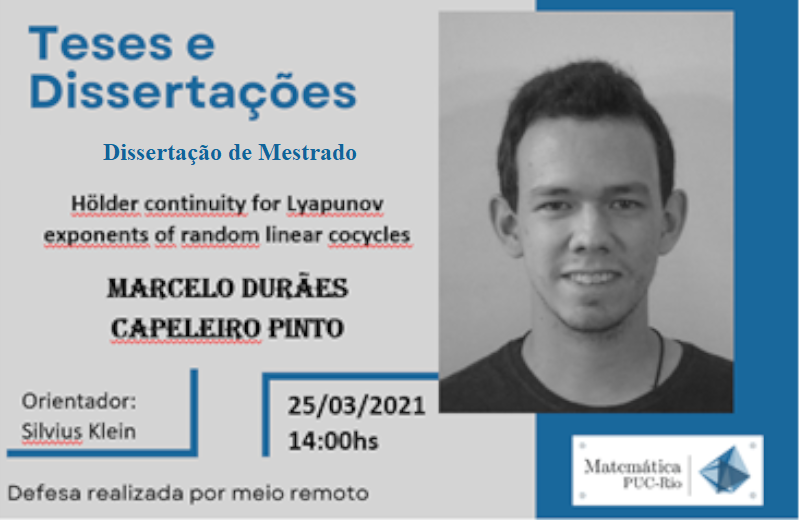Defesa de Tese - Marcelo Durães Capeleiro PintoHölder continuity for Lyapunov exponents of random linear cocycles

A compactly supported probability measure on a group of matrices determines a sequence of i.i.d. random matrices. Consider the corresponding multiplicative process and its geometric averages. Furstenberg-Kesten's theorem, the analogue of the law of large numbers in this setting, ensures that the geometric averages of this multiplicative process converge almost surely to a constant, called the maximal Lyapunov exponent of the given measure. This concept can be reformulated in the more general context of ergodic theory using random linear cocycles over the Bernoulli shift.

A natural question concerns the regularity properties of the Lyapunov exponent as a function of the data. Under an irreducibility condition and in a specific setting (which was later generalized by various authors) Le Page established the Hölder continuity of the Lyapunov exponent. Recently, Baraviera and Duarte obtained a direct and elegant proof of this type of result. Their argument uses Furstenberg's formula and the regularity properties of the stationary measure.

Following their approach, in this work we obtain a new result showing that under the same irreducibility hypothesis, the Lyapunov exponent depends Hölder continuously on the measure, relative to the Wasserstein metric, thus generalizing the result of Baraviera and Duarte .